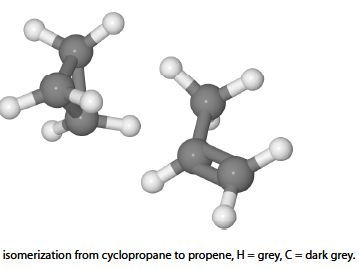Chemistry

# Kinetics and Isomerization: Mathematical Example

In the Lindemann chemical kinetic treatment of the isomerization of molecule A to its isomer B, as in the isomerization reaction of cyclopropane to propene, by below Figure, we have

A ≈ BThe double full-arrows indicate that the reactions have not yet come to equilibrium. During the algebraic manipulation the following kinetic equation is obtained.

K1 [A]2 – k2 [A*] [A] – k3 [A*] = 0

where A* is a reaction intermediate which is chemically different from A and is roughly “half way” between the structures of A and B. The Lindemann mechanism of isomerization will be covered later on in your Chemistry lectures. The square brackets indicate concentrations of the different molecules A and A* involved and for clarity the units have not been shown. Rearrange the equation to get [A*] the concentration of the reaction intermediate as the subject.

Solution

K1 [A]2 – k2 [A*] [A] – k3 [A*] = 0

The intermediate concentration [A*] is obtained as the subject by: (1) moving the term which does not involve [A*] to the right hand side by subtracting k1 [A]2 from both sides; (2) multiplying throughout by —1 to make all three terms positive; (3) taking [A*] outside the two terms on the left hand side; and finally (4) dividing left and right by (k2 [A] + k3) and cancelling out top and bottom of any single term.We cannot cancel out the variable [A] top and bottom in the final equation because the denominator has a plus sign in it (the same would be true if it had had a minus sign) and so the denominator consists of two separate terms, not one single term. You can show this is true by examining the numeric example below.

2 / (2*3 +3) = 2/9      2 / (2*3 +3) ≠ 1/6